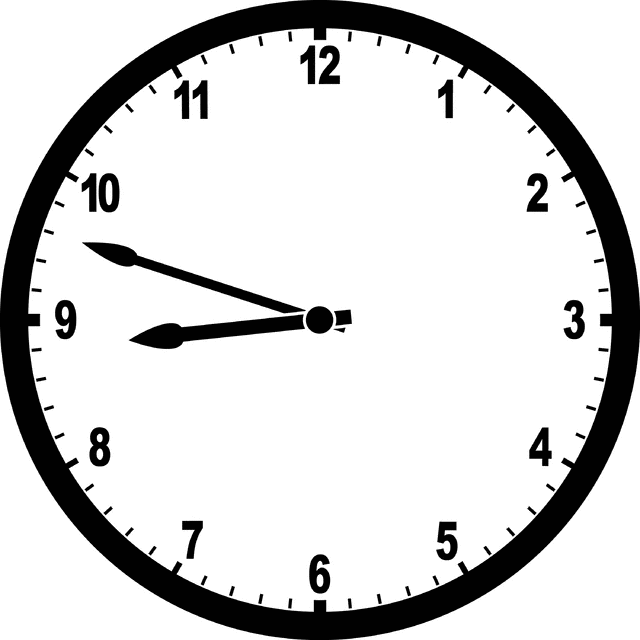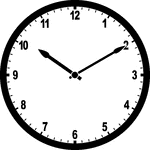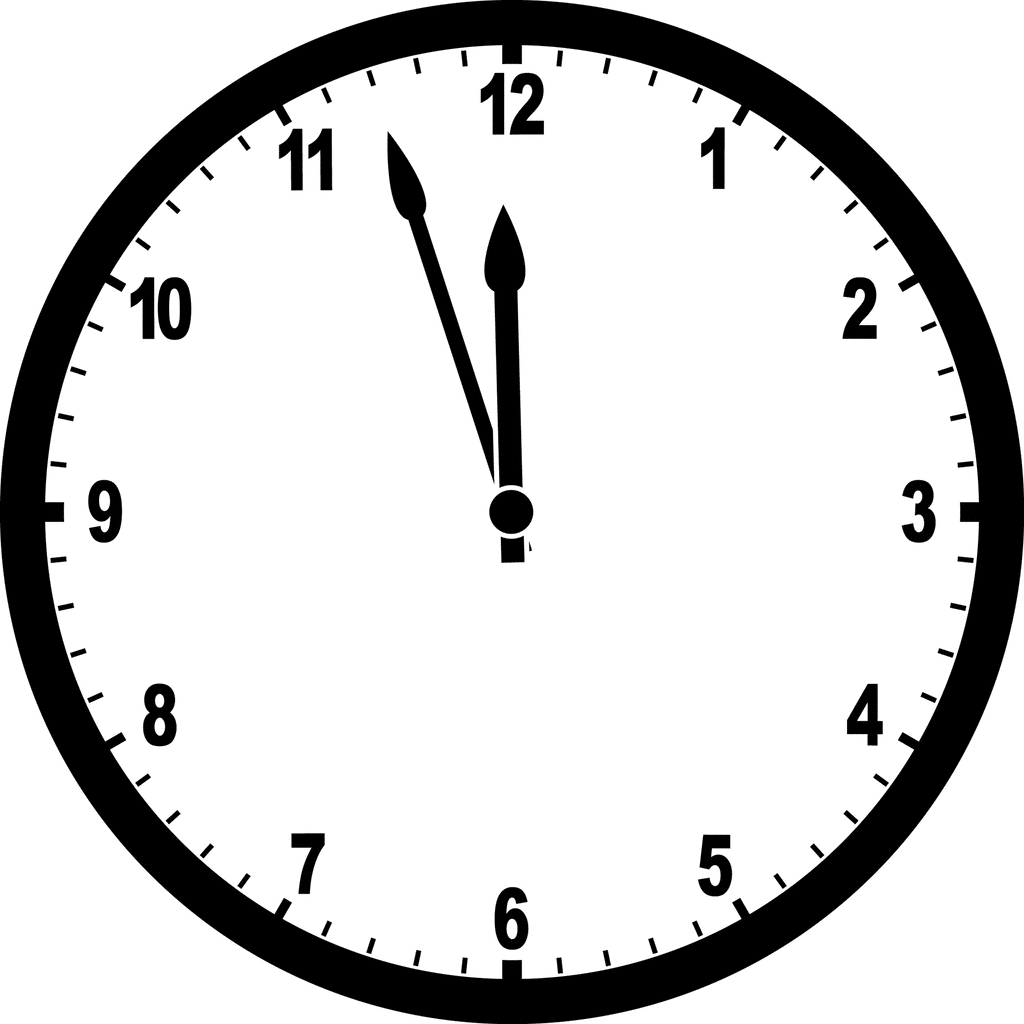Telling Time
Converting Hours and Minutes to Minutes
Rounding
Elapsed Time/Measurement
100

Alia has two dogs that weigh the same amount. Her dogs both weigh 347kg. How much do both her dogs weigh altogether?

347 + 347 = 694kg

100

What time does this clock show?8:48

100

How many total minutes is 1 hour and 34 minutes?

60 + 34 = 94 minutes

100

Round 143 to the nearest HUNDRED

100

100

What unit of measurement would you use to weigh a white rhino?

Kilograms, Grams, or Liters?

Kilograms

200

What is the difference between 442 and 138?

442 - 138 = 304

200

What time does the clock show?1:35

200

How many total minutes is 1 hour and 46 minutes?

60 + 46 = 106 minutes

200

Round 567 to the nearest TEN

570

200

What unit of measurement would you use to measure hot chocolate?

Kilograms, Grams, or Liters?

Liters

300

What number will make this equation true?

300 + ___ = 350 + 40

90

300

What time does this clock show?10:10

300

How many minutes is 1 hour and 59 minutes?

60 + 59 = 119 minutes

300

Round 994 to the nearest TEN

990

300

What is the elapsed time?

Start time: 5:30

End time: 6:47

1 hour 47 minutes

400

Find the sum of 345 and 267

345 + 267 = 612

400

What time does this clock show?12:50

400

How many minutes is in 2 hours and 32 minutes?

60 + 60 + 32 = 152 minutes
400
Round 250 to the nearest HUNDRED

300

400

Jake is working on his homework. He started the working at 3:21. He finished it up at 5:07. How long was he working on his homework?

1 hour 46 minutes

500

Spot the slip-up, then tell the correct answer:

456 - 229 = 133

They forgot to borrow in the ones place, the answer is 127.

500

What time does this clock show?11:57

500

How many minutes in 5 hours?

60 x 5 = 300 minutes

500

Round 1,234 to the nearest TEN

1,230

500

Gandalf the Grey needs to get to Mordor by 8:00pm. If he leaves at 6:26pm, and the trip takes 1 hour and 14 minutes, will he make it in time?

Yes. He would arrive at 7:40pm so he would have 20 more minutes until 8:00pm

Click to zoom